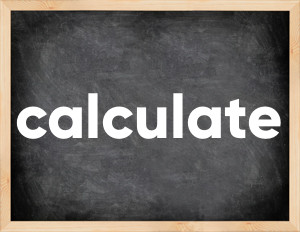# Calculate past tenseThe English verb 'calculate' is pronounced as [ˈkælkjʊleɪt].
Related to: regular verbs.
3 forms of verb calculate: Infinitive (calculate), Past Simple - (calculated), Past Participle - (calculated).

## Here are the past tense forms of the verb calculate

👉 Forms of verb calculate in future and past simple and past participle.
❓ What is the past tense of calculate.

## Calculate: Past, Present, and Participle Forms

Base Form Past Simple Past Participle
calculate [ˈkælkjʊleɪt]

calculated [ˈkælkjʊleɪtɪd]

calculated [ˈkælkjʊleɪtɪd]

## What are the 2nd and 3rd forms of the verb calculate?

🎓 What are the past simple, future simple, present perfect, past perfect, and future perfect forms of the base form (infinitive) 'calculate'?

### Learn the three forms of the English verb 'calculate'

• the first form (V1) is 'calculate' used in present simple and future simple tenses.
• the second form (V2) is 'calculated' used in past simple tense.
• the third form (V3) is 'calculated' used in present perfect and past perfect tenses.

## What are the past tense and past participle of calculate?

The past tense and past participle of calculate are: calculate in past simple is calculated, and past participle is calculated.

### What is the past tense of calculate?

The past tense of the verb "calculate" is "calculated", and the past participle is "calculated".

### Verb Tenses

Past simple — calculate in past simple calculated (V2).
Future simple — calculate in future simple is calculate (will + V1).
Present Perfect — calculate in present perfect tense is calculated (have/has + V3).
Past Perfect — calculate in past perfect tense is calculated (had + V3).

### calculate regular or irregular verb?

👉 Is 'calculate' a regular or irregular verb? The verb 'calculate' is regular verb.

## Examples of Verb calculate in Sentences

•   Will you calculate this for me? (Future Simple)
•   He calculated the approximate number of cases (Past Simple)
•   I have never calculated such a complex formula (Present Perfect)
•   She calculated this surprisingly fast (Past Simple)
•   Calculate this and write down the asnwer (Present Simple)
•   He calculates all of his actions (Present Simple)
•   She calculated that the stock was underprices (Past Simple)
•   We calculated that our trip will cost around a thousand dollars (Past Simple)
•   The result was calculated without adjustments for external factors (Past Simple)
•   Rent is calculated on a monthly basis (Present Simple)

Along with calculate, words are popular clear out and plug up.

Verbs by letter: , , , , , , , , , , , , , , , , , , , , , , , , .